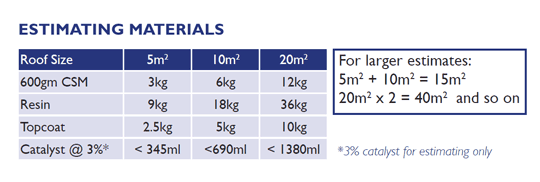# 2. EstimatingThere are several ways to estimate your materials:

• The Short Introduction to Fibreglass Roofing has a table showing quantities of resin, topcoat, catalyst and 600gm mat required for three areas of roofing: 5m2 -10m2- 20m2. Simply combine the quantities shown to obtain the quantities you need eg. 10m2 + 20m2 = 30m2
• You can use our online calculator.

Alternatively you can apply this simple formula:

• Total weight of glass x 3 = weight of Premium Resin required
• Total area (m2) x 0.5 = weight of Premium Topcoat required
• Total weight of topcoat (kg) + resin(kg) x 2% = weight of catalyst required (adjust % as required – see Catalyst Addition Chart)

Worked example for a 6m x 3m garage roof.

• Area = 6m x 3m = 18m2 + 10% wastage = 20m2
• Weight of glass = 20m2 x 600g CSM = 12kg
• Weight of resin = weight of glass (12kg) x 3 = 36kg
• Weight of topcoat = 20m2 x 0.5 = 10kg
• Weight of catalyst @2% = (wt of resin+topcoat) 46kg x 2% = approx 1kg

When calculating the quantity of each trim style remember trims are supplied in 3m lengths